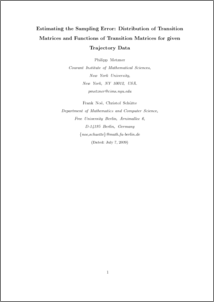Repository: Freie Universität Berlin, Math Department

# Estimating the Sampling Error: Distribution of Transition Matrices and Functions of Transition Matrices for Given Trajectory Data

Metzner, Ph. and Noé, F. and Schütte, Ch. (2009) Estimating the Sampling Error: Distribution of Transition Matrices and Functions of Transition Matrices for Given Trajectory Data. Phys. Rev. E, 80 (2). 021106.Preview
PDF - Accepted Version
Available under License Creative Commons Attribution Non-commercial.

452kB

Official URL: http://dx.doi.org/10.1103/PhysRevE.80.021106

## Abstract

The problem of estimating a Markov transition matrix to statistically describe the dynamics underlying an observed process is frequently found in the physical and economical sciences. However, little attention has been paid to the fact that such an estimation is associated with statistical uncertainty, which depends on the number of observed transitions between metastable states. In turn, this induces uncertainties in any property computed from the transition matrix, such as stationary probabilities, committor probabilities, or eigenvalues. Assessing these uncertainties is essential for testing the reliability of a given observation and also, if possible, to plan further simulations or measurements in such a way that the most serious uncertainties will be reduced with minimal effort. Here, a rigorous statistical method is proposed to approximate the complete statistical distribution of functions of the transition matrix provided that one can identify discrete states such that the transition process between them may be modeled with a memoryless jump process, i.e., Markov dynamics. The method is based on sampling the statistical distribution of Markov transition matrices that is induced by the observed transition events. It allows the constraint of reversibility to be included, which is physically meaningful in many applications. The method is illustrated on molecular dynamics simulations of a hexapeptide that are modeled by a Markov transition process between the metastable states. For this model the distributions and uncertainties of the stationary probabilities of metastable states, the transition matrix elements, the committor probabilities, and the transition matrix eigenvalues are estimated. It is found that the detailed balance constraint can significantly alter the distribution of some observables.

Item Type: Article Mathematical and Computer Sciences > Mathematics Department of Mathematics and Computer Science > Institute of MathematicsDepartment of Mathematics and Computer Science > Institute of Mathematics > Comp. Molecular BiologyDepartment of Mathematics and Computer Science > Institute of Mathematics > BioComputing Group 17 Admin Administrator 03 Jan 2009 20:20 03 Mar 2017 14:39

Repository Staff Only: item control page